# Everything you need to know about Maximum Power Transfer

In this blog we will review the maximum amount of power transferred from a source to a load. Sometimes efficiency, in a circuit, is not the essential/key parameter. We will often overlook how inefficient a special purpose circuit may be in cases where we want it to perform a specific task. An everyday example of this would be an amplifier delivering power to a speaker.  Efficiency may not be as important as getting maximum power to the speaker.  Electrically, “Maximum power transfer” occurs when the internal resistance of the source device is equal to the resistance of the load device.

A basic illustration of Power Transfer: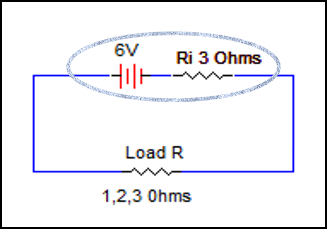In this simple circuit, we have a variety of loads, (1 ohm, 2 ohms, 3 ohms etc.) that can be connected across a 6 volt source with a constant internal resistance of 3 ohms.  When we plot each of these resistors vs. the power they would dissipate in this circuit, it will show that we have maximum power delivered to the load when we have 3 ohms of load resistance.  That is the same value as the amount of internal resistance present in the source.  This is fundamental to maximum power transfer between the source and the load.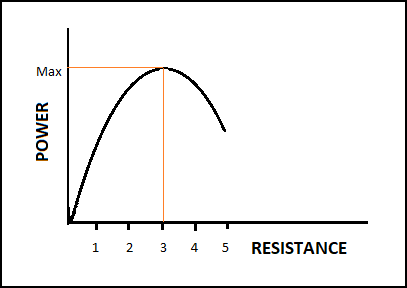The Maximum Power Transfer Theorem:

The Maximum Power Transfer theorem states that, to obtain maximum external power from a source with a finite internal resistance, the resistance of the load must equal the resistance of the source as viewed from its output terminals.

A practical application example using Maximum Power Transfer Theorem:

Say we have an amplifier with an internal resistance of 128 ohms and we want to use this as a source to drive an 8 ohm speaker and allow for maximum power to be passed on to the speaker.  In a practical setting, this would most likely be accomplished by way of a matching transformer. Our task is to determine the matching transformer turns ratio that will provide us with a match between the 128 ohm internal resistance, and the 8 ohm output speaker.  We start by understanding that we need the reflected load seen on the primary side of the transformer (how the load looks to the source), to equal the internal resistance value of the amplifier (source) which was given as 128 ohms.We start with the basic relationship for a reflected load when using a transformer and rearrange and solve for the transformers turns ratio.  We then move on and solve for the turns ratio for this question.
Given:   RP = reflected load, RL = load speaker,  a = transformers turns ratio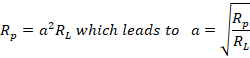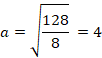This means that we can get maximum power transfer between the amplifier and speaker if we use a matching transformer with a turns ratio of 4:1.  When that transformer is used, the reflected load, which is the load seen by the source on the primary side of the transformer will equal the 128 ohm internal resistance of the amplifier.  This turns ratio that we’ve calculated makes the reflected load appear as 128 ohms to the source which has the same internal resistance.

NOTE:  If you are interested in how the Rp (reflected load value) relationship is derived, it is based on the general power statement that primary power equals secondary power assuming 100 % efficiency, so we have…..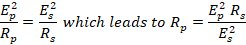Substitute the turns ratio relationship   a=EpEs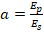into the above reflected load equation giving us the equation used for the reflected load in the amplifier example above, we rearranged this relationship to solve for turns ratio to answer the question: Rp=a2Rs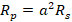We hope this has been helpful to you as a Technician or a student entering the field. If you have any questions about the Electronics or the Electromechanical Technician programs you can reach one of our Program Consultants toll-free at 1-888-553-5333 or by email at info@gbctechtraining.com.